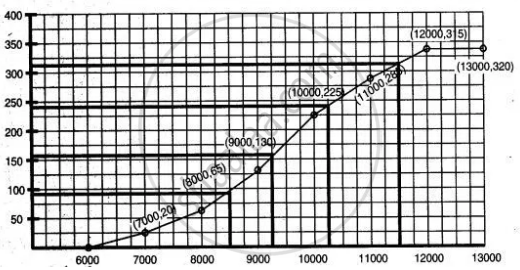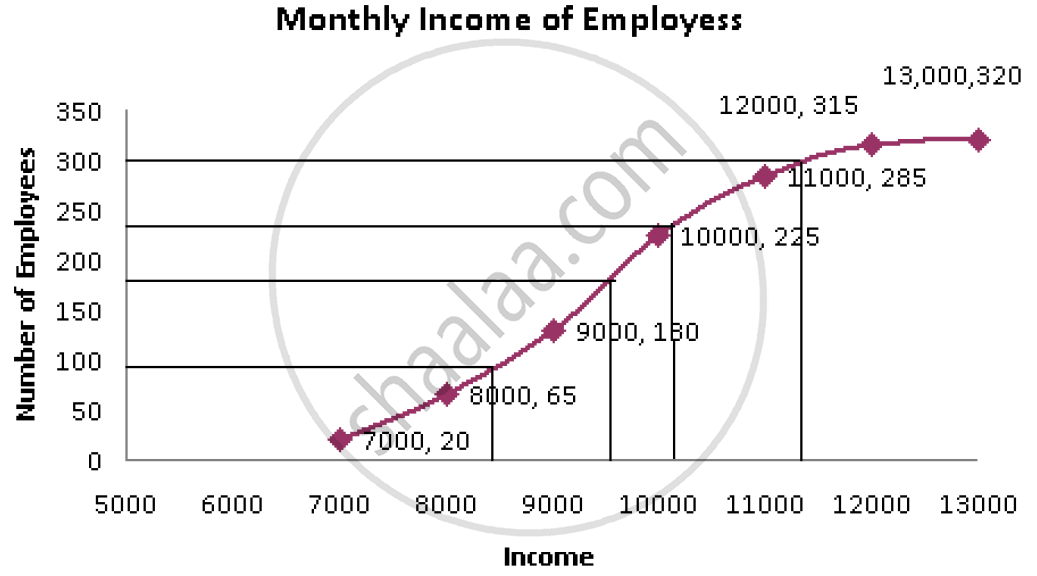Share

# The Monthly Income of a Group of 320 Employees in a Company is Given Below: Draw an Ogive the Given Distribution on a Graph Sheet Taking 2 Cm = Rs. 1000 on One Axis and 2 Cm = 50 Employees on the - Mathematics

Course

#### Question

The monthly income of a group of 320 employees in a company is given below:

 Monthly Income No. of Employees 6000-7000 20 7000-8000 45 8000-9000 65 9000-10000 95 10000-11000 60 11000-12000 30 12000-13000 5

Draw an ogive the given distribution on a graph sheet taking 2 cm = Rs. 1000 on one axis and 2 cm = 50 employees on the other axis. From the graph determine:
(1) the median wage
(2) the number of employees whose income is below Rs. 8500.
(3) if the salary of a senior employee is above Rs. 11,500, find the number of senior employees in the company.
(4) the upper quartile.

#### Solution 1

 Monthly No. of Employees c.f. 6000 - 7000 20 20 7000 - 8000 45 65 8000 - 9000 65 130 9000 - 10000 95 225 10000 - 11000 60 285 11000 - 12000 30 315 12000 - 13000 5 320(i) From the graph, the median wage = 160·5 (appro.)
(ii) The number of employees whose income is below ₹8500 = 90 (appro.)
(iii) The number of senior employees whose salary is above ₹ 11500 = 20 (appro.)
(iv) The upper quartile Q3 = 240 (appro.)

#### Solution 2

 Monthly Income No. of Employees c.f. 6000-7000 20 20 7000-8000 45 65 8000-9000 65 130 9000-10000 95 225 10000-11000 60 285 11000-12000 30 315 12000-13000 5 320Here, n = 320

1) Media  =  n/2 th term = 160 th term

From the graph, the corresponding x coordinate is 9400

median wage = 9400 approx

2) The number of employees whose income is below Rs. 8500 = 95 (approx.)

3) The number of senior employees whose salary is above Rs. 11500 = 320 – 305 = 15 (approx.)

4) The upper quartile Q_3 = ((3n)/4)^"th" term = 240 th term

From the graph, the corresponding x co-ordinate is 10,300 (approx.)

Is there an error in this question or solution?